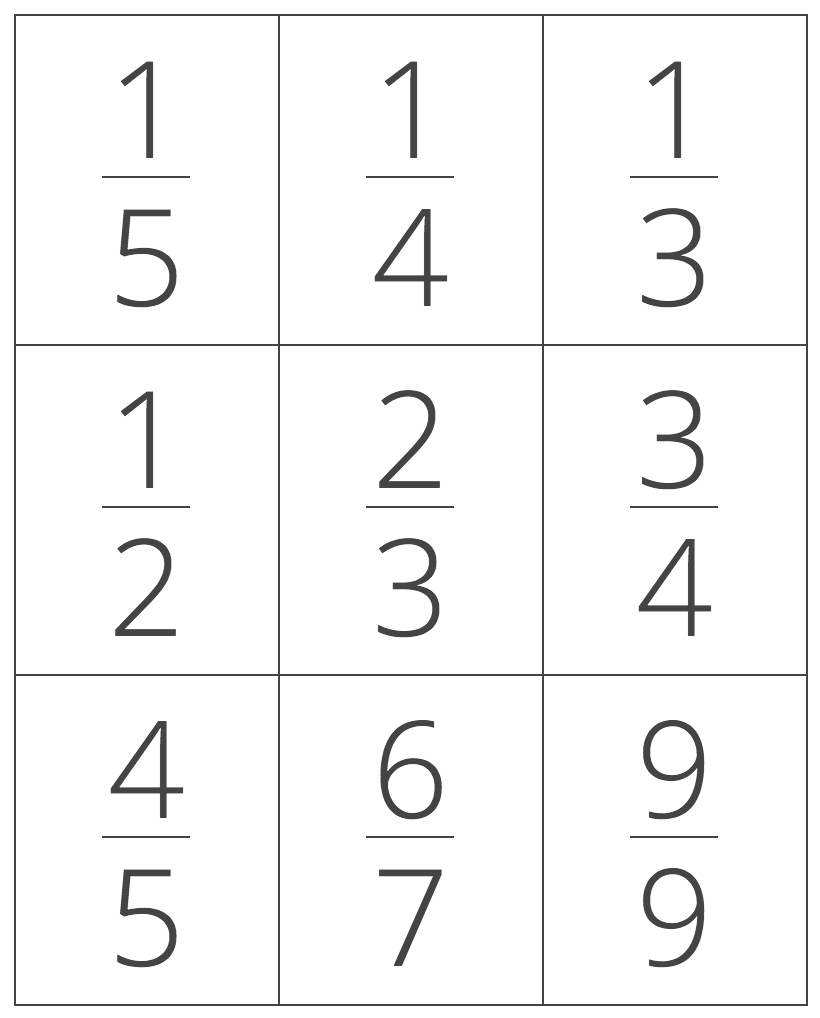# Order Numbers Calculator – Sort Least to Greatest

Sort a list of fractions, decimals, and percent numbers from least to greatest or greatest to least by entering the unsorted list and selecting the sort order. Follow along to see the steps used to sort the list.

Hint: separate numbers using a comma (,)
Sort By:

## Solution:

3 / 5 < 75% < 2.25 < 2 5 / 8

### Steps to Sort Numbers

Step One: convert each number to the equivalent decimal value

 3/5 2 5/8 2.25 75% 0.6 2.625 2.25 0.75

Step Two: sort the list of decimals from least to greatest

 3/5 75% 2.25 2 5/8 0.6 < 0.75 < 2.25 < 2.625

Step Three: rewrite the list using the original values

3 / 5 < 75% < 2.25 < 2 5 / 8
Learn how we calculated this below

## How to Sort Numbers from Least to Greatest

The easiest way to sort a list of fractions, decimals, and percentage values from least to greatest or vice-versa is to start by converting each number to a decimal value.

After converting to decimal, sort the list in ascending or descending order by comparing each decimal value.

The final step is to rewrite the sorted list of decimals using the original form.

## How to Sort a Set of Fractions

Ordering a set of fractions might seem intimidating, but it’s possible to sort the list using a few different methods.### Method One: Order Fractions by Converting to the Same Denominator

The first method allows sorting a list of fractions in just a few simple steps.

First, convert any mixed numbers to a fraction. If you are comparing whole numbers, then convert the whole number to a fraction by placing the number over 1.

The next step is to find the least common denominator for all of the fractions. This is the number that all of the denominators will fit evenly into. If you’re not sure how to do this, check out this handy calculator.

After you have the least common denominator, convert each fraction to the equivalent fraction with that denominator. To do this, divide the least common denominator by the fraction’s denominator.

Then multiply this number by the fraction’s numerator to find the converted numerator. Place the converted numerator over the least common denominator to get the converted fraction.

It is now possible to sort the converted fractions by simply ordering the numerators from small to large.

### Method Two: Order Fractions by Converting to Decimal

A more straightforward method of ordering fractions is to convert each fraction to a decimal, then order them. You can use a calculator for this, but if you’re unsure how, you can try our fraction to decimal calculator.

After all of the fractions are in decimal form, order the decimals from small to large.